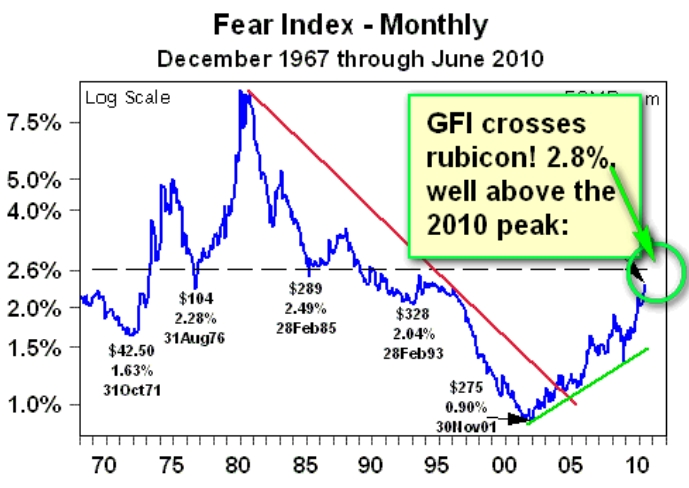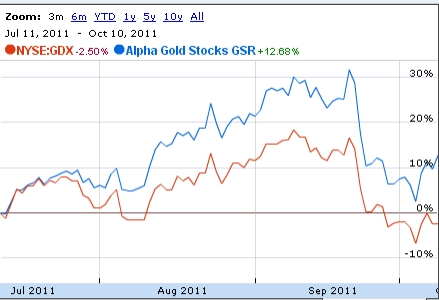©Gold & Silver Fear Indexes Current Gold Fear Index (GFI) August 16, 2012 Chris G. Waltzek A discussion with James Turk this week renewed my interest in his Fear Index. So I decided to calculate the current figure. Given the 2.8% GFI figure listed in the equation above, clearly the gold market mania phase is only beginning, when compared to the 1980's peak:(Courtesy of GoldMoney.com) GFI Formula (click links for data sources): GFI = Total US Gold Reserve Oz. * Gold Price / M3 = GFI = 261,500,000 * \$1,600 / 14,750,000,000,000 = 2.8% Next we use the formula to extrapolate a gold price forecast. The chart shows the Fear Index apogee of roughly 9% in 1980. So we plug in a new gold price of \$5,000 which results in 9% GFI reading. GFI = Total US Gold Reserve Oz. * Gold Price / M3 = GFI = 261,500,000 * \$5,000 / 14,750,000,000,000 = 9% Thus according to the GFI, the fair value for gold is \$5,000. Keep in mind that the gold price forecast moves higher as the M3 money supply figure increases. For instance, if we assume the M3 climbs to the number listed below, in accordance with expectations, gold could soar above \$5,000, to \$7,000: GFI = Total US Gold Reserve Oz. * Gold Price / M3 = GFI = 261,500,000 * \$7,000 / 20,000,000,000,000 = 9%   NEW: Current Silver Fear Index (SFI) Updated: September 13, 2012 Formula (click links for data sources): The GFI index can be used to determine the fair value of silver, by simply using the gold/silver ratio. Assuming a GFI figure of \$5,000 and a gold/silver ratio of 50, the expected silver price is: \$5,000 / 50 = \$100. However, to derive a more accurate silver estimation, we apply the GFI formula to the silver market, resulting with a new indicator: the silver fear index (SFI). Since the government has sold off the strategic silver stockpile, all three billion ounces and the US Mint must purchase silver from the free market, the silver market balance figures produced by the CPM Group are used. This figure represents the annual silver inventories. First we calculate the SFI for the 1980's peak price of \$50 to use as a base for common size measurement: .54%. Next we calculate the current SFI: .04%. Clearly the silver price is EXTREMELY undervalued relative to 1980. But precisely how undervalued is silver, relative to the 1980's peak? SFI = 1980 Total Silver Market Balance Oz. * Silver Price / M3 = SFI = 210,000,000 * \$50 / 1,935,100,000,000 = .54% SFI = 2012 Total Silver Market Balance Oz. * Silver Price / M3 = SFI = 200,000,000 * \$30 / 14,750,000,000,000 = .04% To determine how undervalued silver is today relative to the previous bull market peak price, the .54% SFI number from the 1980 calculation is substituted into the 2012 equation: SFI = 2012 Total Silver Market Balance Oz. * Silver Price / M3 = SFI = 200,000,000 * \$Silver? / 14,750,000,000,000 = .54% SFI = 200,000,000 * \$Silver? = .54% *14,750,000,000,000 SFI = 200,000,000 * \$Silver? = 79650000000 SFI = \$Silver? = 79650000000 / 200,000,000 SFI = Silver = \$398.25 NEW: Therefore, the SFI indicates that silver must climb to nearly \$400 in order to reach it's current fair value. Anecdotally, this is precisely the silver target I proposed in Wealth Building Strategies (2010). Nevertheless, the \$400 price is likely overstated due to the fact that gold has not been included in the calculations. Next the GFI and SFI indexes are combined and adjusted by gold relative to silver (50 = 49/50 = .98) and silver relative to gold (1/50 = .02) resulting with a current silver estimate: Silver FV = GFI (\$5,000) + SFI (\$398) = \$5,398 * Silver:Gold (1/50) = \$108 Gold FV = GFI (\$5,000) + SFI (\$398) = \$5,398 * Gold:Silver(49/50) = \$5290 So investors should expect silver to climb to \$108 and gold to \$5,398 per ounce. Although the numbers listed above are close approximations, for a more accurate calculation of the gold and silver fair value estimates, the GFI and SFI formulas must be combined within the original M3 money supply computation. This article is dynamic - the formulas will be updated after this semester is over. Please click this link for the Housing Fear Index (HFI): click here. Alpha Gold Stocks Beats the XAU/GDX +20% Click Below: